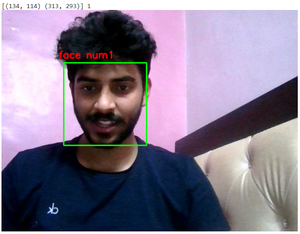Related Articles

# Count number of Faces using Python – OpenCV

• Last Updated : 06 Jun, 2021

Prerequisites: Face detection using dlib and openCV

In this article, we will use image processing to detect and count the number of faces. We are not supposed to get all the features of the face. Instead, the objective is to obtain the bounding box through some methods i.e. coordinates of the face in the image, depending on different areas covered by the number of the coordinates, number faces that will be computed.

### Required libraries:

• OpenCV library in python is a computer vision library, mostly used for image processing, video processing, and analysis, facial recognition and detection, etc.
• Dlib library in python contains the pre-trained facial landmark detector, that is used to detect the (x, y) coordinates that map to facial structures on the face.
• Numpy is a general-purpose array-processing package. It provides a high-performance multidimensional array object and tools for working with these arrays.

Below is the step-wise approach to Count the Number of faces:

Step 1: Import required libraries.

## Python3

 `# Import libraries``import` `cv2``import` `numpy as np``import` `dlib`

Step 2: Open the default camera to capture faces and use the dlib library to get coordinates.

## Python3

 `# (0) in VideoCapture is used to``# connect to your computer's default camera``cap ``=` `cv2.VideoCapture(``0``)``# Get the coordinates``detector ``=` `dlib.get_frontal_face_detector()`

Step 3: Count the number of faces.

• Capture the frames continuously.
• Convert the frames to grayscale(not necessary).
• Take an iterator i and initialize it to zero.
• Each time you get the coordinates to the face structure in the frame, increment the iterator by 1.
• Plot the box around each detected face along with its face count.

## Python3

 `while` `True``:` `    ``# Capture frame-by-frame``    ``ret, frame ``=` `cap.read()``    ``frame ``=` `cv2.flip(frame, ``1``)` `    ``# Our operations on the frame come here``    ``gray ``=` `cv2.cvtColor(frame, cv2.COLOR_BGR2GRAY)``    ``faces ``=` `detector(gray)` `    ``# Counter to count number of faces``    ``i ``=` `0``    ``for` `face ``in` `faces:``        ``x, y ``=` `face.left(), face.top()``        ``x1, y1 ``=` `face.right(), face.bottom()``        ``cv2.rectangle(frame, (x, y), (x1, y1), (``0``, ``255``, ``0``), ``2``)` `        ``# Increment the iterartor each time you get the coordinates``        ``i ``=` `i``+``1` `        ``# Adding face number to the box detecting faces``        ``cv2.putText(frame, ``'face num'``+``str``(i), (x``-``10``, y``-``10``),``                    ``cv2.FONT_HERSHEY_SIMPLEX, ``0.7``, (``0``, ``0``, ``255``), ``2``)``        ``print``(face, i)` `    ``# Display the resulting frame``    ``cv2.imshow(``'frame'``, frame)`

Step 4: Terminate the loop.

## Python3

 `# Enter key 'q' to break the loop``if` `cv2.waitKey(``1``) & ``0xFF` `=``=` `ord``(``'q'``):``    ``break`

Step 5: Clear windows.

## Python3

 `# When everything done, release``# the capture and destroy the windows``cap.release()``cv2.destroyAllWindows()`

Below is the complete program of the above approach:

## Python3

 `# Import required libraries``import` `cv2``import` `numpy as np``import` `dlib`  `# Connects to your computer's default camera``cap ``=` `cv2.VideoCapture(``0``)`  `# Detect the coordinates``detector ``=` `dlib.get_frontal_face_detector()`  `# Capture frames continuously``while` `True``:` `    ``# Capture frame-by-frame``    ``ret, frame ``=` `cap.read()``    ``frame ``=` `cv2.flip(frame, ``1``)` `    ``# RGB to grayscale``    ``gray ``=` `cv2.cvtColor(frame, cv2.COLOR_BGR2GRAY)``    ``faces ``=` `detector(gray)` `    ``# Iterator to count faces``    ``i ``=` `0``    ``for` `face ``in` `faces:` `        ``# Get the coordinates of faces``        ``x, y ``=` `face.left(), face.top()``        ``x1, y1 ``=` `face.right(), face.bottom()``        ``cv2.rectangle(frame, (x, y), (x1, y1), (``0``, ``255``, ``0``), ``2``)` `        ``# Increment iterator for each face in faces``        ``i ``=` `i``+``1` `        ``# Display the box and faces``        ``cv2.putText(frame, ``'face num'``+``str``(i), (x``-``10``, y``-``10``),``                    ``cv2.FONT_HERSHEY_SIMPLEX, ``0.7``, (``0``, ``0``, ``255``), ``2``)``        ``print``(face, i)` `    ``# Display the resulting frame``    ``cv2.imshow(``'frame'``, frame)` `    ``# This command let's us quit with the "q" button on a keyboard.``    ``if` `cv2.waitKey(``1``) & ``0xFF` `=``=` `ord``(``'q'``):``        ``break`  `# Release the capture and destroy the windows``cap.release()``cv2.destroyAllWindows()`

Output:Attention geek! Strengthen your foundations with the Python Programming Foundation Course and learn the basics.

To begin with, your interview preparations Enhance your Data Structures concepts with the Python DS Course. And to begin with your Machine Learning Journey, join the Machine Learning – Basic Level Course

My Personal Notes arrow_drop_up